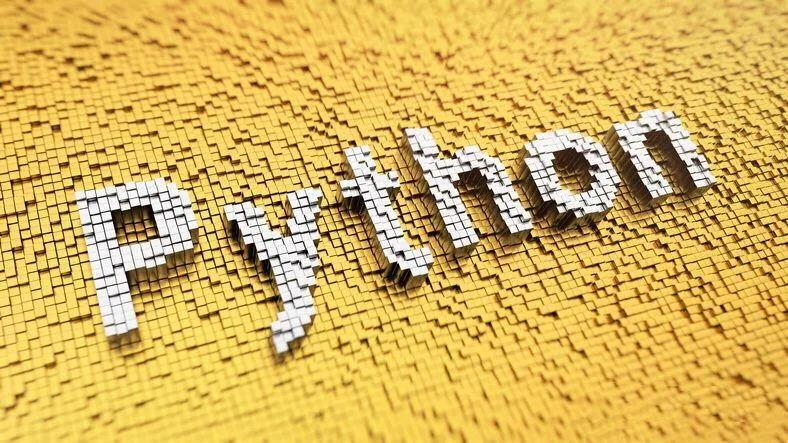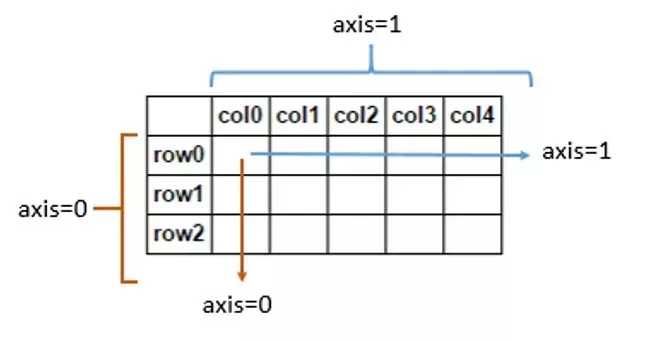# pandas apply 应用套路详解## 基本语法：

DataFrame.apply(func, axis=0, raw=False, result_type=None, args=(), **kwds)

## 基本参数

• func : function

应用到每行或每列的函数。

• axis ：{0 or 'index', 1 or 'columns'}, default 0

函数应用所沿着的轴。

0 or index : 在每一列上应用函数。

1 or columns : 在每一行上应用函数。

• raw : bool, default False

确定行或列以 Series 还是 ndarray 对象传递。

False : 将每一行或每一列作为一个 Series 传递给函数。

True : 传递的函数将接收ndarray 对象。如果你只是应用一个 NumPy 还原函数，这将获得更好的性能。

• result_type : {'expand', 'reduce', 'broadcast', None}, default None

这些只有在 axis=1（列）时才会发挥作用。

expand : 列表式的结果将被转化为列。

reduce : 如果可能的话，返回一个 Series，而不是展开类似列表的结果。这与 expand 相反。

broadcast : 结果将被广播到 DataFrame 的原始形状，原始索引和列将被保留。

• args : tuple

除了数组/序列之外，要传递给函数的位置参数。

**kwds

作为关键字参数传递给函数的附加关键字参数。

## 应用示例

>>> import pandas as pd>>> import numpy as np>>> df = pd.DataFrame([[4, 9]] * 3, columns=['A', 'B'])>>> df   A  B0  4  91  4  92  4  9

>>> df.apply(np.sqrt)     A    B0  2.0  3.01  2.0  3.02  2.0  3.0

>>> df.apply(np.sum, axis=0)A    12B    27dtype: int64

>>> df.apply(np.sum, axis=1)0    131    132    13dtype: int64>>> df.apply(lambda x: [1, 2], axis=1)0    [1, 2]1    [1, 2]2    [1, 2]dtype: object

>>> df.apply(lambda x: [1, 2], axis=1, result_type='expand')   0  10  1  21  1  22  1  2

>>> df.apply(lambda x: pd.Series([1, 2], index=['foo', 'bar']), axis=1)   foo  bar0    1    21    1    22    1    2

>>> df.apply(lambda x: [1, 2], axis=1, result_type='broadcast')   A  B0  1  21  1  22  1  2

## 自定义函数应用框架

### 基本应用

# 制定自定义函数计算逻辑>>> def fx(x):...     return x * 3 + 7...# 应用自定义函数>>> df.apply(fx)    A   B0  19  341  19  342  19  34

### 某列应用函数

>>> df['B'].apply(fx)0    341    342    34Name: B, dtype: int64

### 某列应用函数并新增列

>>> df['new'] = df['B'].apply(fx)>>> df   A  B   new0  4  9  341  4  9  342  4  9  34

### 使用列表推导式应用自定义函数

>>> df['new2'] = [x * 3 + 7 for x in df['B']]>>> df   A  B   new  new20  4  9   34    341  4  9   34    342  4  9   34    34

-- END --## 评论